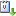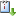Usted está aquí: Computation and Certification of Ramsey-Type Numbers

# Computation and Certification of Ramsey-Type Numbers

Ponente: Jack Wesley
Institución: University of California, Davis
Tipo de Evento: Investigación
Cuándo 06/06/2022 de 12:00 a 13:00 ZOOM ID 882 9372 3602vCaliCal
In this talk we present methods from algebraic geometry and SAT solving to study Ramsey-type numbers. The classical Ramsey number R(r,s) is the smallest integer n such that every 2-coloring of the edges of K_n contains either a clique of size r in the first color or a clique of size s in the second color. Ramsey numbers are notoriously difficult to compute, and few exact values are known. We discuss both computation and verification of these numbers.

First, we look at Rado numbers (a type of Ramsey number): for a positive integer k and equation E, the Rado number R_k(E) is the smallest number n such that every k-coloring of {1,2,...,n} contains a monochromatic solution to E. We compute many new exact values for Rado numbers of the form R_3(ax+by+cz = 0) using SAT solvers. In particular, we give a method for computing infinite families of Rado numbers.

Second, regarding verification: Suppose someone tells you the value of R(50,50). How can one certify that this value is correct? We encode the problem of verifying Ramsey numbers as a system of polynomial equations and show that the degrees of Nullstellensatz certificates for this system are bounded above by the so-called restricted online Ramsey numbers.

This work is partly joint with Yuan Chang and Jesús A. De Loera.